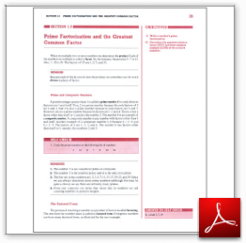Algebra Math Book - Introductory Algebra Thank you for your continual support.

 The power of mathematics rests in the logic of thinking.ID: Sec1-5
Description: Prime and Composite Numbers-Factored Forms-Prime Factorization-GCF and LCM
Price: .99

# Algebra Math book - Introductory Algebra - Chapter 1 - Section 5 - Prime Factorization and the Greatest Common Factor

## Section 1.5 - Objectives

9.  Write a number's prime factorization.

10.  Determine the greatest common factor (GCF) and the least common multiple (LCM) of two or more numbers.

Navigate to
Previous Section or Next Section
or Chapter 1 Details or Other Chapters

This section of my algebra math book, Introductory Algebra, also includes in the download:

• Cover Sheet